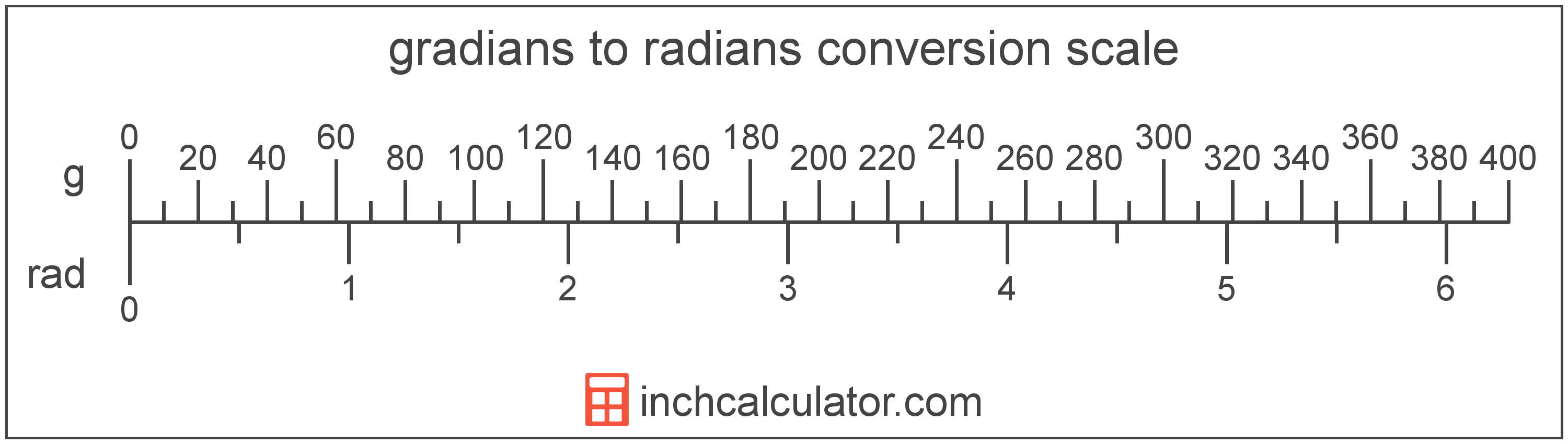Enter the angle in radians below to get the value converted to gradians. The calculator supports values containing decimals, fractions, and π: (π/2, 1/2π, etc)Since one radian is equal to 63.661977 gradians, you can use this simple formula to convert:

The angle in gradians is equal to the angle in radians multiplied by 63.661977.

For example, here's how to convert 5 radians to gradians using the formula above.There are 63.661977 gradians in a radian, which is why we use this value in the formula above.

A radian is the measurement of angle equal to the length of an arc divided by the radius of the circle or arc. 1 radian is equal to 180/π degrees, or about 57.29578°. There are about 6.28318 radians in a circle.

The radian is the SI derived unit for angle in the metric system. Radians can be abbreviated as rad, and are also sometimes abbreviated as c, r, or R. For example, 1 radian can be written as 1 rad, 1 c, 1 r, or 1 R.

Radians are often expressed using their definition. The formula to find an angle in radians is θ = s/r, where the angle in radians θ is equal to the arc length s divided by the radius r. Thus, radians may also be expressed as the formula of arc length over the radius.

Radians are also considered to be a "unitless" unit. That is, when multiplying or dividing by radians, the result does not include radians as part of the final units.

For example, when determining the length of an arc for a given angle, we use the formula above, rearranged to be s = θr. If θ is in radians and r is in meters, then the units of s will be meters, not radian-meters. If θ were in degrees, however, then s would have units of degree-meters.

A gradian is equal to 1/400 of a revolution or circle, or 9/10°. The grad, or gon, is more precisely defined as π/200, or 1.570796 × 10-2 radians.

This unit simplifies the measurements of right angles, as 90° is equal to 100 gradians.

A gradian is sometimes also referred to as a grad, gon, or grade. Gradians can be abbreviated as g, and are also sometimes abbreviated as gr or grd. For example, 1 gradian can be written as 1g, 1 gr, or 1 grd.

In the expressions of units, the slash, or solidus (/), is used to express a change in one or more units relative to a change in one or more other units.